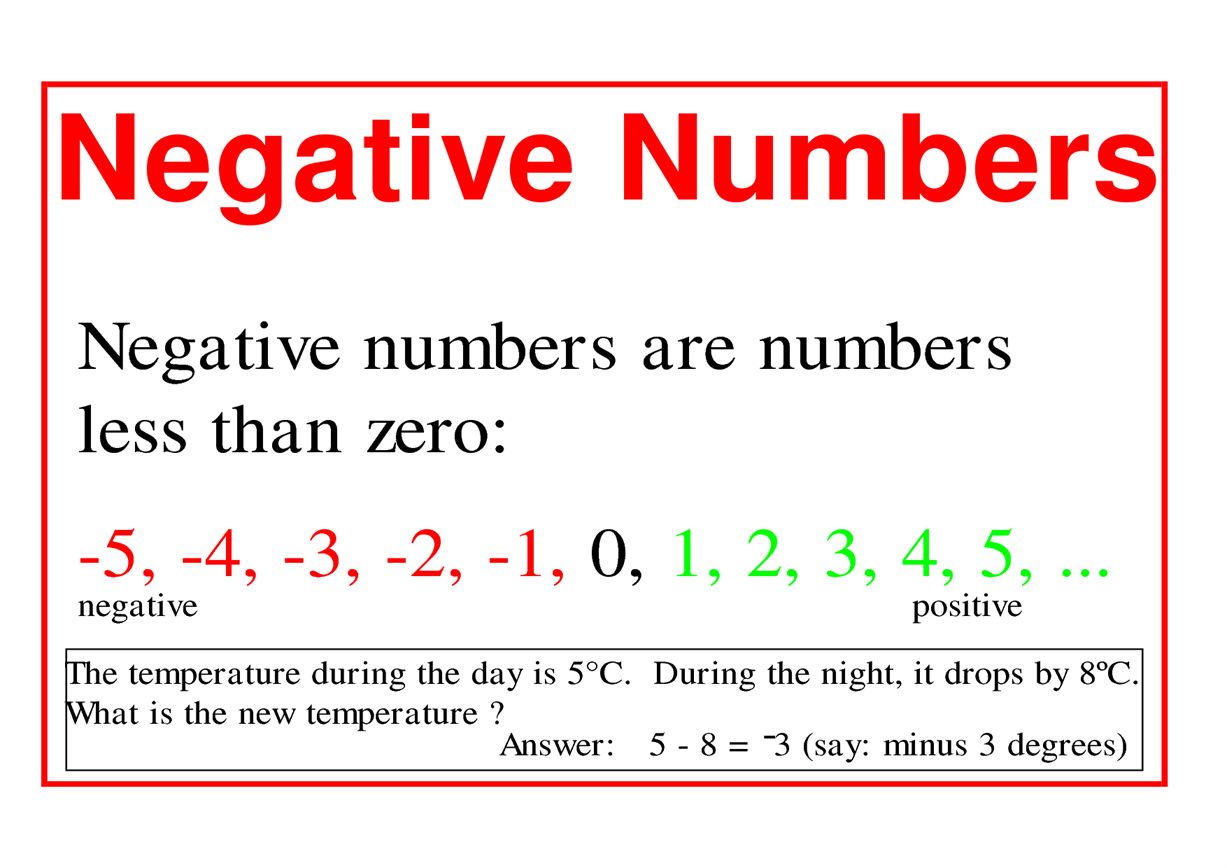# How Negative Numbers Arise?

We have seen some numbers have  a minus sign. If we take a real life instance, the temperature in some region or country is represented as -5˚C or voltage of battery represented by -4V. Let’s see, what does this minus sign indicates and how  is it related to the number system.According to mathematicians, negative numbers are those numbers whose value is less than zero. Also, they are enlisted with the real numbers. These numbers have been interpreted in many ways. Some of the interpretations are as follows:

• A negative number is a number that is less than zero
• The opposite of a positive number
• They are numbers represented to the left of zero on a number line
• The amount of loss or absence
• A directed quantity

## History of Negative Numbers

In ancient times, negative numbers were considered incorrect and people thought that they were not possible. In history, Nine Chapters on the Mathematical Art takes the credit  of the first book which mentioned about negative numbers. Later, by the 7th century, even Indians started to use these numbers. The arithmetical operations and rules for negative numbers which helped to solve mathematical problems were developed by Islamic mathematicians.

In the modern era, negative numbers are considered as a part of real numbers. All real numbers are either a positive or a negative number. Zero is an exception. Negative numbers belong to the class of real numbers which also include zero and positive numbers. These numbers have wide application in life incidents such as in science, sports, finance and few more.

### Use of Negative Numbers Around the House

Let us see the following example to have a better insight:

Pavan wanted to buy a notebook which was marked at Rs. 20. But he had only Rs.12.  The shopkeeper lent him the notebook and mentioned Rs.8 as balance in bill.

But how did he know that he has to take from or  give money to Pavan? This situation could be better understood with help of positive and negative numbers.

The numbers with positive sign repesents credit and negative sign represents debit. Therefore, some numbers are represented with a plus sign while some with negative sign. Numbers which are symbolized with plus sign are called positive numbers, whereas numbers with minus sign are called as negative numbers. Negative numbers are additive inverse of positive numbers. This means that when a positive number is added to a negative number with same magnitude it results into zero.  Therefore, profit can be denoted by a plus sign (+) and loss can be  denoted by a minus sign (-). For example, + 2  is a positive number while -2 is a negative number.

To solve problems on the topic, download Byju’s – The Learning App from Google Play Store and watch interactive videos. Also, take free tests to practice for exams. To study about other topics, visit www.byjus.com and browse among thousands of interesting articles.